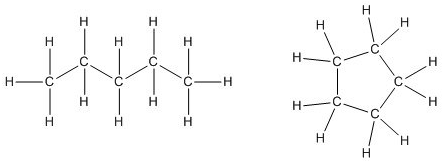# 3.2: Straight-Chain Alkanes

•• Contributed by Allison Soult
• Senior Lecturer (Chemistry) at University of Kentucky

Learning Outcomes

• Distinguish between organic and inorganic compounds.
• Describe features of alkane structure.
• Pair each prefix with its numerical definition.
• Distinguish between an alkane and a cycloalkane.

## Organic Compounds

All living things on earth are formed mostly of carbon compounds. The prevalence of carbon compounds in living things has led to the epithet “carbon-based” life. The truth is we know of no other kind of life. Early chemists regarded substances isolated from organisms (plants and animals) as a different type of matter that could not be synthesized artificially, and these substances were thus known as organic compounds. The widespread belief called vitalism held that organic compounds were formed by a vital force present only in living organisms. The German chemist Friedrich Wohler was one of the early chemists to refute this aspect of vitalism, when, in 1828, he reported the synthesis of urea, a component of many body fluids, from nonliving materials. Since then, it has been recognized that organic molecules obey the same natural laws as inorganic substances, and the category of organic compounds has evolved to include both natural and synthetic compounds that contain carbon. Some carbon-containing compounds are not classified as organic, for example, carbonates and cyanides, and simple oxides, such as CO and CO2. Although a single, precise definition has yet to be identified by the chemistry community, most agree that a defining trait of organic molecules is the presence of carbon as the principal element, bonded to hydrogen and other carbon atoms. Organic compounds often contain other elements such as nitrogen, oxygen, sulfur, or phosphorus. The number of carbon and hydrogen atoms in an organic compound is usually much greater than the number of other atoms.Figure $$\PageIndex{1}$$: All organic compounds contain carbon and most are formed by living things, although they are also formed by geological and artificial processes. (credit left: modification of work by Jon Sullivan; credit left middle: modification of work by Deb Tremper; credit right middle: modification of work by “annszyp”/Wikimedia Commons; credit right: modification of work by George Shuklin)

Today, organic compounds are key components of plastics, soaps, perfumes, sweeteners, fabrics, pharmaceuticals, and many other substances that we use every day. The value to us of organic compounds ensures that organic chemistry is an important discipline within the general field of chemistry. The element carbon gives rise to a vast number and variety of compounds that are found in biological and industrial settings. These compounds typically contain functional groups which include atoms other than carbon and hydrogen. A particular arrangement of atoms within a compound is known as a functional group. Types of functional groups will be discussed in the next chapter. For now, we will identify organic and inorganic (which means not organic) compounds and look at structures for the simplest of organic compounds, hydrocarbons.

### Hydrocarbons

A hydrocarbon is an organic compound that is made up of only carbon and hydrogen. A hydrocarbon is the simplest kind of organic molecule and is the basis for all other more complex organic compounds. Hydrocarbons can have single, double, or triple bonds between the carbon atoms. Alkanes will be discussed here and other hydrocarbons will be covered later.

### Alkanes

An alkane is a hydrocarbon in which there are only single covalent bonds. The simplest alkane is methane, with the molecular formula $$\ce{CH_4}$$. The carbon is the central atom and makes four single covalent bonds to hydrogen atoms.Figure $$\PageIndex{2}$$: Methane is the simplest hydrocarbon and is shown with a structural formula, a ball-and-stick model, and a space-filling model.

The next simplest alkane is called ethane $$\left( \ce{C_2H_6} \right)$$ and consists of two carbon atoms with a single covalent bond between them. Each carbon is then able to bond to three hydrogen atoms. The alkane series progresses from there, increasing the length of the carbon chain by one carbon at a time. Structural formulas for ethane, propane $$\left( \ce{C_3H_8} \right)$$, and butane $$\left( \ce{C_4H_{10}} \right)$$ are shown below.These alkanes are called straight-chain alkanes because the carbon atoms are connected in one continuous chain with no branches. Naming and writing structural and molecular formulas for the straight-chain alkanes is straightforward. The name of each alkane consists of a prefix that specifies the number of carbon atoms and the ending -ane. The molecular formula follows the pattern of $$\ce{C_{n}H_{2n + 2}}$$ where $$\ce{n}$$ is the number of carbons in the chain. The table below lists the first ten members of the alkane series.

Table $$\PageIndex{1}$$: First Ten Members of the Alkane Series
Name Molecular Formula Condensed Structural Formula
Methane $$\ce{CH_4}$$ $$\ce{CH_4}$$
Ethane $$\ce{C_2H_6}$$ $$\ce{CH_3CH_3}$$
Propane $$\ce{C_3H_8}$$ $$\ce{CH_3CH_2CH_3}$$
Butane $$\ce{C_4H_{10}}$$ $$\ce{CH_3CH_2CH_2CH_3}$$
Pentane $$\ce{C_5H_{12}}$$ $$\ce{CH_3CH_2CH_2CH_2CH_3}$$
Hexane $$\ce{C_6H_{14}}$$ $$\ce{CH_3CH_2CH_2CH_2CH_2CH_3}$$
Heptane $$\ce{C_7H_{16}}$$ $$\ce{CH_3CH_2CH_2CH_2CH_2CH_2CH_3}$$
Octane $$\ce{C_8H_{18}}$$ $$\ce{CH_3CH_2CH_2CH_2CH_2CH_2CH_2CH_3}$$
Nonane $$\ce{C_9H_{20}}$$ $$\ce{CH_3CH_2CH_2CH_2CH_2CH_2CH_2CH_2CH_3}$$
Decane $$\ce{C_{10}H_{22}}$$ $$\ce{CH_3CH_2CH_2CH_2CH_2CH_2CH_2CH_2CH_2CH_3}$$

Note that the table shows a variation of a structural formula called a condensed structural formula, which we will discuss later. In this formula, the covalent bonds are understood to exist between each carbon and the hydrogens associated with it, as well as between carbon atoms.

### Cycloalkanes

Alkanes can also exist in a ring structure which is known as a cycloalkane. For example, pentane is a chain of five carbon atoms while cyclopentane is a ring of five carbons as shown in the figure below.Figure $$\PageIndex{3}$$: Structures of pentane (left) and cyclopentane (right).